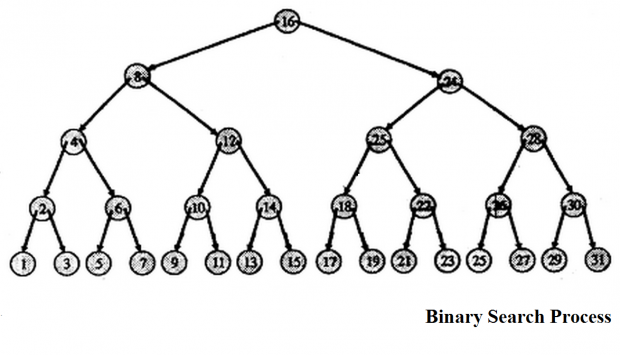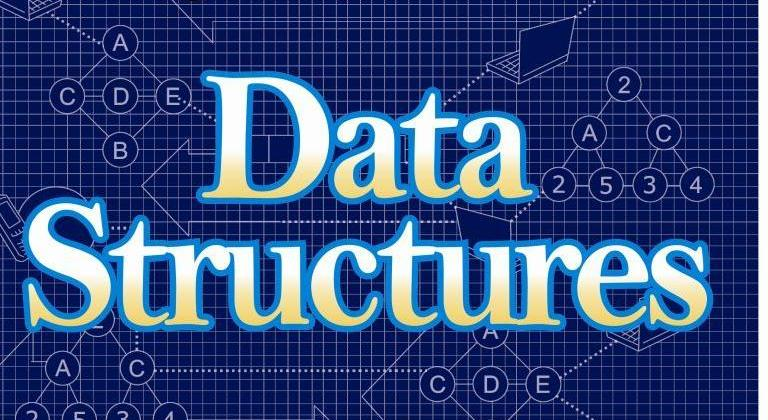# Binary Search Algorithm In Data StructureNilanchala   |   #C Programming   |   Sep 17, 2023,

In this tutorial, we will see binary search algorithm In data structure. Before we reading through Binary search algorithm, let us recap sequential search or linear search. In Linear search algorithm searching begins with searching every element of the list till the required record is found. Also it doesn’t demand the sequence or order of elements in the list. If the list is quite huge, then this approach is not optimal. The drawbacks of sequential search can be eliminated by using Binary search algorithm.

## How binary search works?

In binary search, it halves the size of the list to search in each iterations. It is faster then Linear search. Binary search requires sorted data to operate on since the data may not be contiguous like the pages of a book. We cannot guess which quarter of the data the required item may be in. So we divide the list in the center each time.

### Example: Search for page number 6 in a book of 20 pages

Typical example of binary search is searching for a page in a book. Suppose you were searching for page 6 in book of 20 pages. You would first open it at random towards the later half of the book. If the page is less than 6, you would open at a page to the right, it is greater than 6 you would open at a page to the left, repeating the process till page 6 was found. As you can see, by the first instinctive search, you dramatically reduced the number of pages to search.

In the above example, we have an sorted list of size 20. The first comparison is with the middle element Page number 10. This eliminates the last 10 elements, as Page 6 is less then 10. The second comparison is with the middle element from Page 1 to Page 10,  i.e. with Page 5. This eliminates Page 1 to Page 5 as Page 6 is greater then Page 5. This continues until Page 6 is found. Now let us formulate the algorithm for binary search.

## Algorithm Binary Search

This represents the binary search method to find a required item in a list sorted in increasing order .

```INPUT: Sorted LIST of size N, Target Value T
OUTPUT: Position of T in the LIST = I
BEGIN

1. MAX = N
MIN = 1
FOUND = false

2. WHILE (FOUND is false) and (MAX > = MIN)
2.1 MID = (MAX + MIN)DIV 2
2.2 If T = LIST [MID]
I=MID
FOUND = true
Else If T < LIST[MID]
MAX = MID-1
Else
MIN = MD+1
END
```

## Analysis of Binary Search

The binary search method needs no; more than [Iog2n] + 1 comparisons. This implies that for an array of a million entries, only about twenty comparisons will be needed. Contrast this with the case of sequential search which on the average will need (n+1)/2 comparisons.

In the binary search method just described above, it is always the key in the middle of the list currently being examined that is used for comparison. The splitting of the list can be illustrated through a binary decision tree in which the value of a node is the index of the key being tested.

Suppose there are 31 records, then the first key compared is at location 16 of the list since (1 + 31)/2 = 16. If the key is less than the key at location 16 the location 8 is tested since (1 + 15)/2 = 8; or if key is less than the key at location 16, then the location 24 is tested.### Example: Program to search for an item using Binary Search

```#include <stdio.h>

int main()
{
int c, first, last, middle, n, search, array;

printf("Enter number of elements\n");
scanf("%d",&n);

printf("Enter %d integers\n", n);

for ( c = 0 ; c < n ; c++ )
scanf("%d",&array[c]);

printf("Enter value to find\n");
scanf("%d",&search);

first = 0;
last = n - 1;
middle = (first+last)/2;

while( first <= last )
{
if ( array[middle] < search )
first = middle + 1;
else if ( array[middle] == search )
{
printf("%d found at location %d.\n", search, middle+1);
break;
}
else
last = middle - 1;

middle = (first + last)/2;
}
if ( first > last )

return 0;
}
```### Nilanchala

I'm a blogger, educator and a full stack developer. Mainly focused on Java, Spring and Micro-service architecture. I love to learn, code, make and break things.

## Related articles2 months ago ·C Programming

### Selection Sort Example In Data Structure

EasyPost2 months ago ·C Programming

### Bubble Sort Example In Data Structure

EasyPost2 months ago ·C Programming

### Insertion Sort Example In Data Structure

EasyPost2 months ago ·C Programming

### Sorting Methods In Data Structures

EasyPost2 months ago ·C Programming

### Sequential Search Algorithm in Data Structure

EasyPost2 months ago ·C Programming

EasyPost